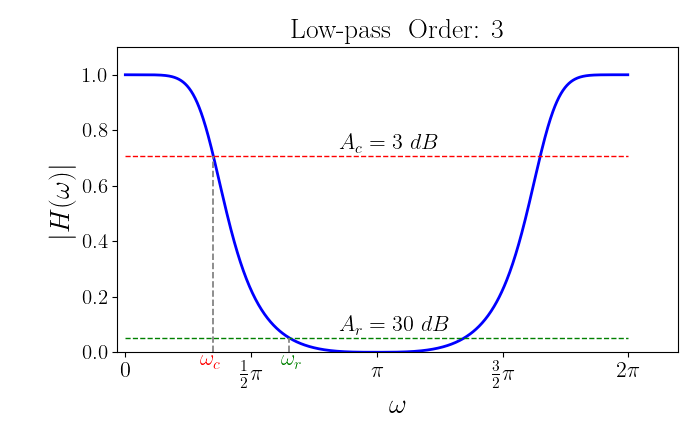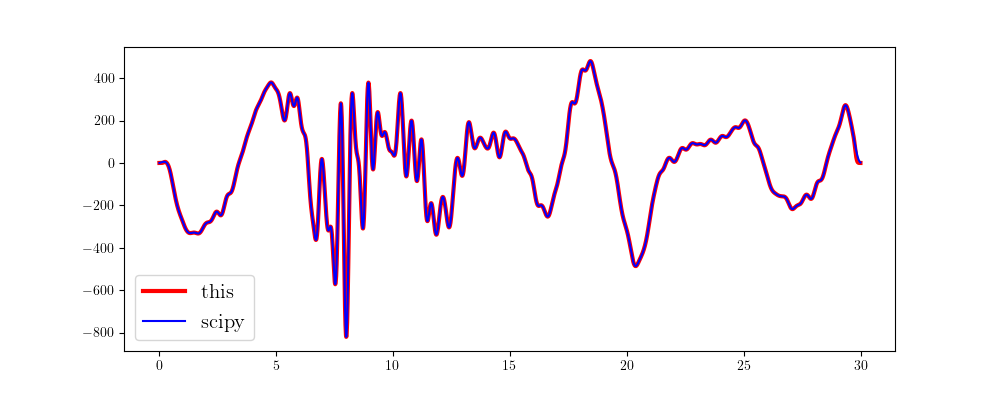#### Butterworth filters

$$|H(w)|^2=\frac{1}{1+( \frac{tan\frac{\omega}{2}} {tan\frac{\omega_c}{2}})^{2N}} \tag{1}$$

#### Low-pass filter

Given the attenuation values $A_c$ and $A_r$ at cut-off frequencies $\omega_c$ and $\omega_r$, respectively, we first calculate the orders $N$ of filter. We know, \begin{aligned} A_r &= -20lg|H(\omega_s)|\\ &= -20lg\frac{1}{\sqrt{1+(\frac{tan\frac{\omega _r}{2}}{\frac{\omega_c}{2}})^{2N}}} \end{aligned} \tag{2}, and the orders $N$ is given by $$N = \frac{1}{2}\frac{lg(10^{\frac{A_r}{10}}-1)}{lg(\frac{tan\frac{\omega_r}{2}}{tan\frac{\omega_c}{2}})} \tag{3}.$$ However, if you give the filter orders $N$, it can control the width of transition band of the filter.

We rewrite eq. $(1)$ as $$|H(\omega)|^2 = \frac{tan^{2N}\frac{\omega_c}{2}}{tan^{2N}\frac{\omega_c}{2}+tan^{2N}{\frac{\omega}{2}}} \tag{4}$$ and according to the $Euler’s$ formula $e^{j\theta}=cos\theta+jsin\theta$, we have \begin{aligned} tan\frac{\omega}{2} &= \frac{sin\frac{\omega}{2}}{cos\frac{\omega}{2}}\\ &= \frac{1}{j}\frac{ e^{j\frac{\omega}{2}}-e^{-j\frac{\omega}{2}} } { e^{j\frac{\omega}{2}}+e^{-j\frac{\omega}{2}} }\\ &= \frac{1}{j}\frac{ 1-e^{-j\omega} } { 1+e^{-j\omega} } \end{aligned} \tag{5}. Let $z=e^{-j\omega}$, and combine eq. $(5)$ to yield $$|H(z)|^2 = \frac{tan^{2N}\frac{\omega_c}{2}} {tan^{2N}\frac{\omega_c}{2}+(-1)^N(\frac{1-z^{-1}}{1+z^{-1}})^N} \tag{6}.$$ We know there are $N$ repeated zeros, namely, $-1$, of this transfer function and we calculate the poles as follows. $$q_k= \frac{1-z_k^{-1}}{1+z_k^{-1}}= \begin{cases} &tan\frac{\omega_c}{2}e^{j\frac{2k+1}{2N}\pi}, \text{ for } N \text{ is even, and }k=0, 1, 2, \cdots, 2N-1;\\ &tan\frac{\omega_c}{2}e^{j\frac{k}{N}\pi}, \text{ for } N \text{ is odd and }k=0, 1, 2, \cdots, 2N-1. \end{cases} \tag{7}.$$ We get $$z_k = \frac{1+q_k}{1-q_k} \tag{8}.$$ Lastly, the transfer function can be calculated by $$H(z) = \frac{1}{2^N}\frac{\prod_{|z_k|<1}(1-z_k)(1+z^{-1})^N}{\prod_{|z_k|<1}(1-z_kz^{-1})} \tag{9}.$$ Here, we separately give attenuation values $3 \ dB$ and $30 \ dB$ at cut-off frequency $\omega_c$ and stop frequency $\omega_r$ to show the frequency response.We give a python code example of implementing a low-pass filter and compare this result with that filtered by the butterworth filter in scipy.

  1 2 3 4 5 6 7 8 9 10 11 12 13 14 15 16 17 18 19 20 21 22 23 24 25 26 27 28 29 30 31 32 33 34 35 36 37 38 39 40 41 42 43 44 45 46 47 48 49 50 51 52 53 54 55  import numpy as np import matplotlib.pyplot as plt from obspy import read from scipy.signal import butter, filtfilt from scipy import fft def lowpass(d, fs, fc, orders=4, zerophase=False, taper=0.01): n = len(d) fd = fft.fft(d) f = np.arange(n) * fs / n w = 2 * np.pi * f / fs wc = 2 * np.pi * fc / fs k = np.arange(2*orders) if orders % 2 == 0: q = np.tan(wc/2) * np.exp(1j*(k+0.5)/orders*np.pi) else: q = np.tan(wc/2) * np.exp(1j*k/orders*np.pi) p = (1 + q) / (1 - q) z = np.exp(1j*w) h = np.ones_like(w, dtype=complex) for pp in p[abs(p)<1]: h *= ((1-pp) / (1-pp/z)) h *= ( (1+1/z) ** orders / 2**orders ) dd = fft.ifft(fd * h).real if zerophase: dd = fft.ifft(fft.fft(dd[::-1])*h)[::-1].real ni = int(n*taper) k1 = np.arange(ni) k2 = (-k1)[::-1] ta1 = np.cos(np.pi*k1/ni/2) ** N ta2 = np.cos(np.pi*k2/ni/2) ** N dd[:ni] *= ta2 dd[-ni:] *= ta1 return f, h, dd tr = read() dt = tr.stats.delta fs = 1 / dt d1 = tr.data.copy() n = len(d1) t = np.arange(n) * dt fc = 2.5 fr = 1.5 Ar = 30 N = 4 f, h, d2 = lowpass(d1, fs, fc, orders=N, zerophase=True, taper=0.01) [b, a] = butter(N, 2*fc/fs, 'lowpass') d3 = filtfilt(b, a, d1) plt.figure(figsize=(10, 5)) plt.plot(t, d2, 'r', lw=3, label='this') plt.plot(t, d3, 'b', lw=1.5, label='scipy') plt.legend(fontsize=15) plt.show()This result perfectly agrees with that in scipy, doesn’t it?